Electron and Ion Optics

The following article is from The Great Soviet Encyclopedia (1979). It might be outdated or ideologically biased.

Electron and Ion Optics

the branch of science that studies the behavior of electron and ion beams in a vacuum under the influence of electric and magnetic fields. Inasmuch as the study of electron beams began before the study of ion beams and the former are used much more extensively than the latter, the narrower term “electron optics” is widely used. Electron and ion optics deals mainly with problems of the formation, focusing, and deflection of charged particle beams and the production, by means of such beams, of optical images on a luminescent screen or photographic film. Such images are commonly called electron-optical and ion-optical images. The development of electron and ion optics has largely been in response to the requirements of electronics technology.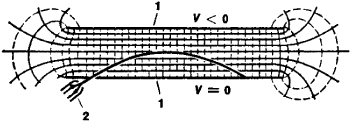Figure 1. Deflection of an electron beam in the uniform field of a parallel-plate capacitor: (1) capacitor plates, (2) electron emitter producing the electron beam. The lines of force are represented by broken lines, and the intersections of the equipotential surfaces and the plane of the figure are represented by solid lines. The field potential V increases from top to bottom.

The rise of electron and ion optics was associated with the creation of the electron-beam tube in the late 19th century. In the first oscilloscope electron-beam tube, constructed by C. F. Braun in 1897, the electron beam was deflected by a magnetic field. Deflection by means of an electrostatic field was accomplished by J. J. Thomson; in his experiments to determine the ratio of the electron’s charge to its mass, Thomson passed a beam through a parallel-plate capacitor located inside an electron-beam tube. In 1899 a coil of insulated wire through which an electric current flowed was used to focus an electron beam in an electron-beam tube. It was not until 1926, however, that the German scientist H. Busch theoretically considered the motion of charged particles in the magnetic field of such a coil; Busch demonstrated that the coil was suitable for producing accurate electron-optical images and thus constituted an electron lens.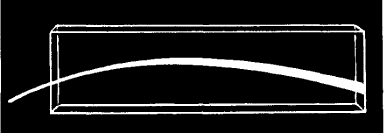Figure 2. Deflection of a beam of light in an inhomogeneous medium. The refractive index n increases from top to bottom. The optical medium is an inhomogeneous mixture of carbon disulfide (n = 1.63) and benzene (n = 1.5) in a transparent vessel. The percent of carbon disulfide in the mixture increases from 0 to 100 percent from the top layers of the liquid to the bottom layers.

The subsequent development of electron lenses (both magnetic and electrostatic) opened the way to the creation of the electron microscope, image converter, and various other devices for the production of accurate electron-optical images of objects that either emit electrons or in some way affect electron beams. The construction of specialized electron-beam tubes for use in television and radar equipment and for data recording, storage, and reproduction led to the further development of the branches of electron and ion optics associated with the control of charged particle beams. The development of equipment for the analysis of electron and ion flows, such as beta spectrometers, mass spectrometers, and other analytical devices, significantly influenced the development of electron and ion optics.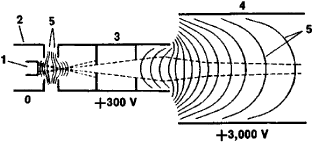Figure 3. Electron-optical system with rotational symmetry, designed to produce an electron beam (an electron gun): (1) indirectly heated cathode, (2) focusing electrode, (3) first anode, (4) second anode, (5) intersections of equipotential surfaces of the electrostatic field and the plane of the figure. Broken lines indicate the beam contours. Shown beside the electrodes are their potentials with respect to the cathode, whose potential is assumed equal to zero. Electrodes (1), (2), and (3) form a cathode lens, and electrodes (3) and (4) form an immersion lens.

Electron and ion optics generally does not consider the problems that arise in microwave technology, and only rarely treats processes occurring in electron tubes, particle accelerators, and other equipment and devices whose specific characteristics place them outside the branch’s primary field of interest.

The solution of most of the problems of electron and ion optics requires consideration only of the motion of charged particles within the framework of classical mechanics, since the wave nature of particles essentially is not manifested in such problems. In such an approximation electron and ion optics is called geometrical electron and ion optics because of the consistent analogy with the geometrical optics of light rays; the analogy is grounded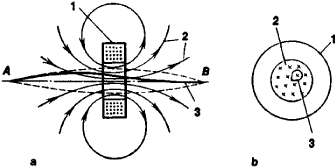Figure 4. Magnetic lens in the form of a toroidal coil: (a) side view, (b) front view; (1) coil, (2) magnetic lines of force, (3) electron trajectory. Broken lines indicate the contours of the electron beam, which emerges from point A (the object) and is focused at point B (the image).

in the similarity between the behavior of charged particle beams in electric and magnetic fields and that of light beams in inhomogeneous optical media. The similarity is apparent from a comparison of Figures 1 and 2. The analogy is underlain by the more general analogy between classical mechanics and the geometrical optics of light that was established by W. R. Hamilton, who proved in 1834 that the general equation of mechanics (the Hamilton-Jacobi equation) is similar in form to the optical eikonal equation. As in the geometrical optics of light, the concept of the refractive index is introduced in geometrical electron and ion optics, and the eikonal method is often used in calculating image errors (aberrations), most of which are analogous to the aberrations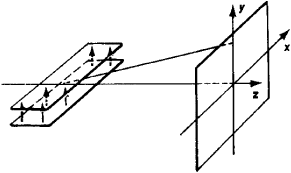Figure 5. Deflection of a beam of positively charged particles in the field of a parallel-plate electrostatic capacitor. The arrows indicate the direction of the electric field inside the capacitor.

of optical systems. When the approximation of geometrical electron and ion optics is inadequate, for example, in the investigation of the resolution of an electron microscope, the techniques of quantum mechanics are used.

Electric and magnetic fields that have rotational (circular) symmetry with respect to the optical axis of a system are used extensively in electron-optical devices. Electron lenses and electron mirrors with such fields are said to be axially symmetric. Electric fields with rotational symmetry are generated by electrodes in the shape of cylinders, cups, and diaphragms with round apertures (Figure 3). Electromagnets (occasionally, permanent magnets) with pole pieces in the form of bodies of revolution or toroidal coils with a winding of insulated wire, through which an electric current is passed, are used to generate axially symmetric magnetic fields (Figure 4).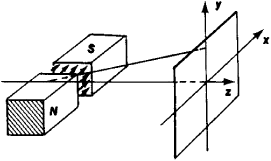Figure 6. Deflection of a beam of positively charged particles by a transverse magnetic field: (N) and (S) magnetic poles. The arrows indicate the direction of the magnetic field in the gap between the poles.

Axially symmetric lenses and mirrors produce accurate electron-optical images if the charged particles travel sufficiently close to the field axis of symmetry and if their initial velocities differ little from one another. If these conditions are not fulfilled, the image errors become significant. When the object and image lie outside the field, axially symmetric electron lenses always constitute collecting lenses. In axially symmetric electron lenses, as in normal optical lenses with spherical surfaces, the image can be only direct or inverted, but in magnetic electron lenses the image additionally may be rotated by some angle.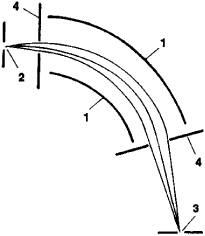Figure 7. Deflection and focusing of a beam of charged particles by a sectional tubular capacitor: (1) capacitor electrodes, (2) exit slit of the source of charged particles, (3) entrance slit of the particle detector, (4) beam-limiting apertures. The electrodes have the shape of sections of circular cylinders. The source slit acts as the object. The divergent particle beam emerges from the source slit with a specific energy and is focused to form a linear image of the source slit; the image is perpendicular to the plane of the figure and aligned with the detector slit.

The electron-optical properties of a field with rotational symmetry are determined by the location of the cardinal points, which are analogous to the cardinal points of axially symmetric optical imaging systems: two foci, two principal points, and two nodal points. The image is constructed according to the rules of geometrical light optics. Axially symmetric electrostatic fields exhibit the same five types of third-order geometrical aberrations as do centered optical systems with spherical surfaces: spherical aberration, astigmatism, curvature of image field, distortion, and coma. Magnetic fields exhibit three additional aberrations: anisotropic distortion, anisotropic astigmatism, and anisotropic coma.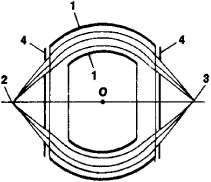Figure 8. Spherical capacitor: (1) capacitor electrodes, (2) point object, (3) image of object, (4) beam-limiting apertures. The electrodes have the shape of sections of two concentric spheres. The image lies on the straight line passing through the source and the center O of the spheres.

Furthermore, there are three types of chromatic aberration (two in electrostatic fields), caused by some unavoidable energy spread of the particles entering the field. In general, the aberrations of fields with rotational symmetry exceed in magnitude, under comparable conditions, the aberrations of centered optical systems; that is, electron lenses and electron mirrors are substantially inferior in quality to optical systems. The compensation for or reduction of aberrations is one of the basic problems in theoretical electron and ion optics.

Other types of electron lenses and mirrors have fields that exhibit different types of symmetry. They form images of point objects as line segments, but sometimes are capable of providing stigmatic focusing (point-to-point focusing) as well. Cylindrical electrostatic and magnetic lenses and mirrors produce line images of point objects. The fields in such electron lenses are “two-dimensional” (their intensities are described by functions of just two Cartesian coordinates) and are symmetric with respect to some central plane near which the charged particles move. Precise focusing in just one direction is needed in many analytical electric vacuum devices. In such cases it is expedient to use transaxial electrostatic electron lenses or transaxial electron mirrors, whose aberrations in the central plane are very small (comparable to the aberrations of optical lenses). Quadrupole electron lenses (electrical and magnetic) are used for work with high-energy charged particle beams.

Electron-optical devices with electric or magnetic fields directed across the beam are used to deflect charged particle beams. The parallel-plate capacitor (Figure 5) represents the simplest electrical deflecting element. In order to reduce the deflecting voltage, systems with electrodes of more complex shape may be used in electron-beam tubes. The magnetic fields used to deflect beams may be generated by electromagnets (Figure 6) or by conductors through which a current flows.

In analytical devices that use deflecting electric and magnetic fields to separate (resolve) charged particles with respect to energy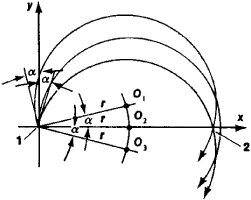Figure 9. Deflection and focusing of a beam of charged particles by a uniform magnetic field: (1) object, (2) image; (O,), (O1), and (O1) centers of the circular trajectories of the particles. The charged particles emitted by a linear object (slit) within the small angle 2α initially diverge and are then focused, describing semicircles of radius r, which is the same for all particles of identical mass and energy. The focused particles form an image of the object in the shape of a strip with a width of rα2. The linear object and strip image are situated parallel to the magnetic lines of force, which are perpendicular to the plane of the figures.

and mass, the forms of the fields used vary widely. Extensive use is also made of the fields’ ability to focus beams. The electric fields are usually formed by various capacitors: parallel-plate, tubular (Figure 7), and spherical types (Figure 8). Of the magnetic fields, uniform fields (Figure 9) and sectoral fields (Figure 10) are often used. The boundaries of sectoral magnetic fields may be bent in order to improve the quality of focusing, and nonuniform magnetic fields whose strength varies according to a specific law may also be used for the same purpose.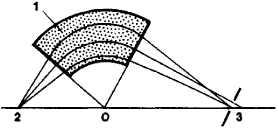Figure 10. Deflection and focusing of a beam of charged particles by a sectoral magnetic field: (1) magnetic field, (2) object (source slit), (3) image. The magnetic lines of force are perpendicular to the plane of the figure. The image lies on the line connecting the object with the vertex O of the sector. The width of the image is of the same order as in a uniform magnetic field.

The deflecting electric and magnetic devices discussed above, which are sometimes called electron (ion) prisms, differ from optical prisms in that they not only deflect but also focus charged particle beams. As a result of focusing, parallel beams that enter the fields of such devices cease to be parallel after deflection. However, the creation of high-quality analytical electron and ion devices, constructed on an exact analogy with optical prism spectrometers, requires electron (ion) prisms that also preserve beam parallelism. Telescopic systems of electron lenses may be used for this function. By adding two electron lenses to an electron prism, with one (the collimator lens) at the inlet and the other (the focusing lens) at the exit, it is possible to construct an analytical device that combines high resolution and high electron-optical transmission.

REFERENCES

Artsimovich, L. A., and S. Iu. Luk’ianov. Dvizhenie zariazhennykh chastits v elektricheskikh i magnitnykh poliakh. Moscow, 1972.
Bonshtedt, B. E., and M. G. Markovich. Fokusirovka i otklonenie puchkov v elektronnoluchevykh priborakh. Moscow, 1967.
Brüche, E., and O. Scherzer. Geometricheskaia elektronnaia optika. Leningrad, 1943. (Translated from German.)
Glaser, W. Osnovy elektronnoi optiki. Moscow, 1957. (Translated from German.)
Grinberg, G. A. Izbrannye voprosy matematicheskoi teorii elektricheskikh i magnitnykh iavlenii. Moscow-Leningrad, 1948.
Zinchenko, N. S. Kurs lektsii po elektronnoi optike, 2nd ed. Kharkov, 1961.
Kel’man, V. M., and S. Ia. Iavor. Elektronnaia optika, 3rd ed. Leningrad, 1968.
Strashkevich, A. M. Elektronnaia optika elektrostaticheskikh sistem. Moscow-Leningrad, 1966.
Iavor, S. Ia. Fokusirovka zariazhennykh chastits kvadrupol’nymi linzami. Moscow, 1968.

V. M. KELMAN and I. V. RODNIKOVA